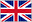# Lecture 08 Syntax Analysis and Parsing

0    11 flashcards

QuestionAnswerwhat is the prime characteristic of a predictive parser
A predictive parser is characterized by its ability to choose the production to apply solely on the basis of the next input symbol and the current nonterminal being processed.
Table-Driven LL(1) Parsing uses what a stack for what? a
 a stack to store the symbols of the right hand side of the productions in right-to-left order so that the leftmost symbol is on the top of the stack.
Table-Driven LL(1) Parsing uses what a parsing table for what? a
A parsing table stores the actions (i.e., the rules) the parser should take based on the input token and what value is on top of the stack
The construction of the parse table requires computing the f___and f___sets of the n_____of the grammar. These sets are sets of t____
The construction of the table requires computing the first and follow sets of the nonterminals of the grammar. These sets are sets of terminals.
The construction of the parse table requires computing the ___and ___sets of the ____of the grammar. These sets are sets of ____
The construction of the table requires computing the first and follow sets of the nonterminals of the grammar. These sets are sets of terminals.
what are the first sets?
The first set of a nonterminal symbol A, written as First(A) is the set of terminals which start the sequences of symbols derivable from A.